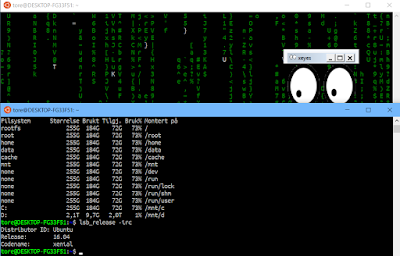## Saturday, 21 October 2017

### X11 Subsystem running WSL Windows 10 subsystem for LinuxThis article will look at running graphical Linux applications in Windows 10. As many of you know, Windows 10 can offer a subsystem for Linux running inside Windows 10. We need to download and install a X11 server to be able to run graphical applications that need more than the console, that is a graphical user interface. First off, download XMing for Windows Server from here:

XMing X Server for Windows Server
After installation of Xming, start Xming from your start button.
Now we need to install the Linux Subsystem itself, if you have not done this yet.
Inside Powershell, enter the following command as an administrator:
```
Enable-WindowsOptionalFeature -Online -FeatureName Microsoft-Windows-Subsystem-Linux

```
If you are not a Windows Insider yet, you must join this program. See this page for a description how to obtain WSL or Windows Subsystem for Linux and install it.
Installation guide of WSL - Windows Subsystem for Linux After you have downloaded WSL and installed it using Powershell, select Launch and in the console Window after WSL is further installed and setup, enter a user in Linux you will use as administrator (in Linux terms, this is the root user). You can add another user using the `adduser` command as root in the Bash console. You can access WSL Linux inside Windows 10 by selecting the menu item `Bash on Ubuntu on Windows`.

### Getting started using WSL

``` apt-get update && apt-get upgrade ``` The version of Linux distro can be found using this command:

```
lsb_release -irc
```

As we see, I am running Ubuntu 16 Xenial. Next off, we are going to support X-Server. Download first the X11 apps.
``` root@tore# apt-cache search x11-apps x11-apps - X applications ```
Install X11-Apps. This will also download all required additional pacages. ``` apt-get install X11-Apps ```
You need to do this as root or switching to a super user and use the sudo command. Next off, edit your ~/.bashrc file. This is similar to the \$Profile file that Powershell uses. You are setting up your environment here to make sure you can use X-Server based Graphical User Interfaces. Such as Gimp, Firefox, Stellarium, Quake and so on - requiring a GUI. But we will start off with running a X11-app, such as Xeyes. Make sure your ~/.bashrc file got the following content. First download Nano if you do not want to use Vim or Vi. (apt-get install nano)
``` nano ~/.bashrc ``` Next off, add the following line at least: ``` export DISPLAY=:0 ``` We can also add more nice colors and some additional information and offer to switch to a non-root user initally.
```export DISPLAY=:0
LS_COLORS='rs=0:di=1;35:ln=01;36:mh=00:pi=40;33:so=01;35:do=01;35:bd=40;33;01:cd=40;33;01:or=40;31;01:su=37;41:sg=30;43:ca=30;41:tw=30;42:ow=34;42:st=37;44:ex=01;32:*.tar=01;31:*.tgz=01;31:*.arj=01;31:*.taz=01;31:*.lzh=01;31:*.lzma=01;31:*.tlz=01;31:*.txz=01;31:*.zip=01;31:*.z=01;31:*.Z=01;31:*.dz=01;31:*.gz=01;31:*.lz=01;31:*.xz=01;31:*.bz2=01;31:*.bz=01;31:*.tbz=01;31:*.tbz2=01;31:*.tz=01;31:*.deb=01;31:*.rpm=01;31:*.jar=01;31:*.war=01;31:*.ear=01;31:*.sar=01;31:*.rar=01;31:*.ace=01;31:*.zoo=01;31:*.cpio=01;31:*.7z=01;31:*.rz=01;31:*.jpg=01;35:*.jpeg=01;35:*.gif=01;35:*.bmp=01;35:*.pbm=01;35:*.pgm=01;35:*.ppm=01;35:*.tga=01;35:*.xbm=01;35:*.xpm=01;35:*.tif=01;35:*.tiff=01;35:*.png=01;35:*.svg=01;35:*.svgz=01;35:*.mng=01;35:*.pcx=01;35:*.mov=01;35:*.mpg=01;35:*.mpeg=01;35:*.m2v=01;35:*.mkv=01;35:*.webm=01;35:*.ogm=01;35:*.mp4=01;35:*.m4v=01;35:*.mp4v=01;35:*.vob=01;35:*.qt=01;35:*.nuv=01;35:*.wmv=01;35:*.asf=01;35:*.rm=01;35:*.rmvb=01;35:*.flc=01;35:*.avi=01;35:*.fli=01;35:*.flv=01;35:*.gl=01;35:*.dl=01;35:*.xcf=01;35:*.xwd=01;35:*.yuv=01;35:*.cgm=01;35:*.emf=01;35:*.axv=01;35:*.anx=01;35:*.ogv=01;35:*.ogx=01;35:*.aac=00;36:*.au=00;36:*.flac=00;36:*.mid=00;36:*.midi=00;36:*.mka=00;36:*.mp3=00;36:*.mpc=00;36:*.ogg=00;36:*.ra=00;36:*.wav=00;36:*.axa=00;36:*.oga=00;36:*.spx=00;36:*.xspf=00;36:';
export LS_COLORS
PS1='\e[37;1m\u@\e[35m\W\e[0m\\$ '
echo "Welcome to Bash on Ubuntu on Windows. To switch to your user Tore :"
echo "su tore && cd /home/tore"
echo "Do this now? [y/n]"

if [ "\$input" = "y" ]; then
cd /home/tore
su tore
ls -al
fi

echo "To adjust profile file edit the file ~\.bashrc with Nano"

```
Last, we can test out everything, running Xeyes. Note that you can test out more advanced Linux applications such as Gimp by running: ``` apt-get install gimp ``` This will install Gimp, the Gnu Image Manipulation Program. Then just enter gimp from the console. You can also run such programs in the background by adding the ampersand (&): gimp &

Sometimes, we need to force an exit of such a Linux app with a GUI running from the console entering Ctrl+C to force exit the process.

## Tuesday, 3 October 2017

### Setting up a Git alias with a shell function

This short article will show you how to search in the log of a Git repository with a shell function. This shell function can also receive a positional parameter to use in the shell function. First off, edit the .gitconfig file in your user's home folder. On Linux Mint for example, the folder should reside in the /home directory. You can use nano for example. (apt-get install nano)

```.gitconfig :

[alias]
searchlog = "!f() { git log --all --decorate --graph -i --grep \"\$1\";  }; f"

```

Note the positonal parameter \$1 here, we escape the quote also. The function inside the alias is a shell function.

```To use this alias command, type for example:
git searchlog test
```
This will search the Git log for the parameter passed in (test) and include some flags to decorate the log displayed. Using Git aliases, we can do lenghty Git commands with shorter aliased commands.